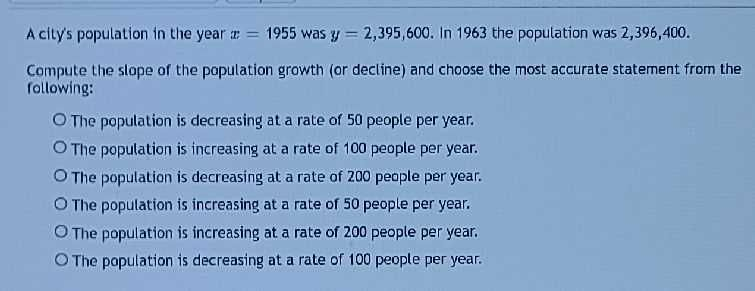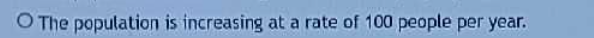### ¿Todavía tienes preguntas de matemáticas?

Pregunte a nuestros tutores expertos
Algebra
PreguntaA city's population in the year $$x = 1955$$ was $$y = 2,395,600$$ . In $$1963$$ the population was $$2,396,400 .$$ Compute the slope of the population growth (or decline) and choose the most accurate statement from the following: The population is decreasing at a rate of $$50$$ people per year. The population is increasing at a rate of $$100$$ people per year. The population is decreasing at a rate of $$200$$ people per year. The population is increasing at a rate of $$50$$ people per year. The population is increasing at a rate of $$200$$ people per year. The population is decreasing at a rate of $$100$$ people per year.